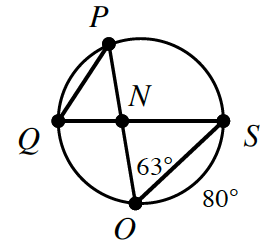### Home > INT2 > Chapter 10 > Lesson 10.2.5 > Problem10-110

10-110.

If $\overline{QS}$ is a diameter and $\overline{PO}$ is a chord of the circle at right, calculate the measure of the geometric parts listed below.

1. $m∠QSO$

Refer back to the Math Notes in Lesson 10.2.4.

1. $m∠QPO$

See part (a).

$50°$

1. $m∠ONS$

Use what you found in part (a) to solve for $m∠ONS$.

1. $m\overarc { P S }$

$126°$

1. $m\overarc{ P Q }$

Half of a circle is equal to $180°$.

1. $m∠PQN$

See the Math Notes for this lesson.

$63°$